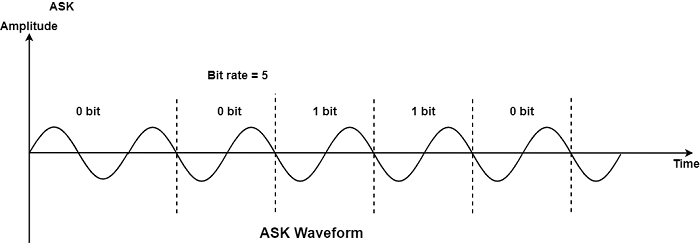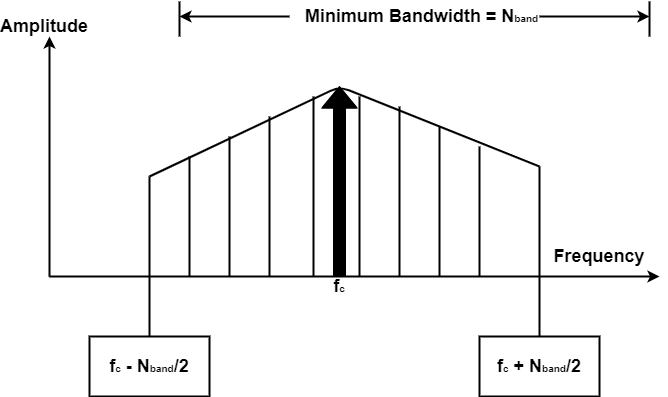# What is Amplitude Shift Keying (ASK) in Computer Network?

Computer NetworkNetworkOperating System

#### Artificial Neural Network and Machine Learning using MATLAB

54 Lectures 4 hours

In amplitude shift keying (ASK), the carrier signal’s strength varies to describe binary 1 or 0. Both frequency and phase remain constant while the amplitude modifies. A bit duration is a time that represents one bit. The signal’s peak amplitude during each bit duration is continuous, and its value is based on the bit (0 or 1). The speed of communication using ASK is defined by the physical features of the transmission medium.

The figure shows a conceptual view of ASK −ASK is hugely susceptible to noise interference. The noise defines unintentional voltages defined onto a line by several phenomena, including heat or electromagnetic induction generated by different sources. These unintentional voltages connect with the signal to convert the amplitude. As 0 can be modified to 1, and a 1 to 0.

A famous ASK method is known as on-off-keying (OOK). In OOK, one of the bit values is described by no voltage. The benefit is a reduction in the amount of energy needed to send data.

## Bandwidth for ASK

The bandwidth of a signal is the total range of frequencies that signal a spectrum of many simple frequencies. The most important ones are those with the carrier frequency, at the middle.

Relationship between band rate and bandwidth in ASK.Bandwidth requirements for ASK are evaluated using the formula.

$$BW=(1+D)\times N_{band}$$

Where,

BW is the bandwidth

$N_{band}$is the band rate.

D is the factor associated with the condition of the line (With the least value of 0).

As we can see, the minimum bandwidth needed for transmission is similar to the band rate.